Next: Equidistant-level model (infinitely many Up: Theoretical models Previous: Strong-pairing limit,

## Degenerate shell: Seniority model

Let us consider the seniority (or pairing quasispin) model [27,28], i.e., the model for N nucleons moving in a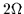-fold degenerate shell described by the seniority-pairing Hamiltonian (14). For this model the exact solution can be written in terms of quasispin quantum numbers [see Eq. (26)]. Alternatively, the ground-state energy can be expressed in terms of the seniority quantum number s (see, e.g.,  p. 222):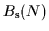=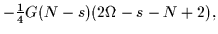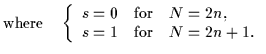(39)

The corresponding value of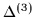is given by: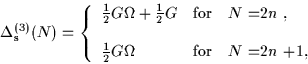(40)

which implies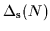=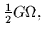(41)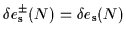= G. (42)

It is seen that, in the seniority model, filters (6) and (7) give the OES values (41) and single-particle energy spacings (42) which are independent of the particle number N. Values of the OES (41) correctly reproduce the exact pairing gap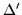=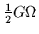, which is defined as a half of the lowest excitation energy in an even system, and which we denote by a prime to distinguish it from the OES. The meaning of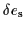is less obvious. It is because the mean-field (Hartree-Fock, HF) treatment of Hamiltonian (14) yields only one 2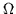-fold degenerate single-particle level at energy -G, while our interpretation ofassumes that only the Kramers degeneracy is present. Nevertheless, one may compare exact values ofwith those obtained in an approximated way and see whether the approximate ground-state energies reproduce features of the mass spectrum represented by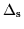and.

In the seniority model, the Hartree-Fock-Bogoliubov (HFB) equations (which in this case are identical with the BCS equations) can be solved analytically. Indeed, the BCS occupation coefficient is given by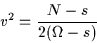(43)

(see e.g.  p. 233), and the ground-state energy is: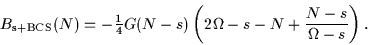(44)

The resulting three-mass filter (1) can be written as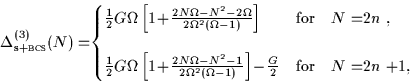(45)

and hence filters (6) and (7) give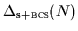=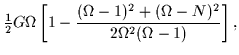(46)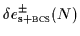=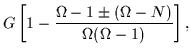(47)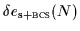=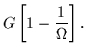(48)

It is seen that in the limit of large(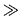1), the BCS approximation (46) reproduces the leading order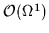of the exact OES result (41). The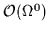deviation from the exact result smoothly depends on N and reaches the minimum (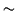G/4) at the middle of the shell (N=).

For the energy-spacing filters the deviations behave as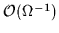. While the symmetric filter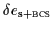does not depend on N, both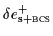and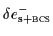vary weakly with the particle number. Namely, at the beginning of the shell (N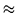0),Gand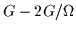, at the middle of the shell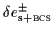=, and at the top of the shell (N)Gand. This behavior follows from a simple identity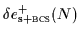=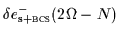reflecting the particle-hole symmetry of the model.

The analysis presented in this section illustrates the advantages of comparing exact and approximate results (similarly as experimental and theoretical results) by looking at appropriate filters. Analytical results available in this model allow the explicit study of pairing and mean-field effects. The high degeneracy of the seniority model does not allow for extracting the energy spacings between the deformed levels; to this end, results for the non-degenerate models are shown in the following sections.Next: Equidistant-level model (infinitely many Up: Theoretical models Previous: Strong-pairing limit,
Jacek Dobaczewski
2000-03-09